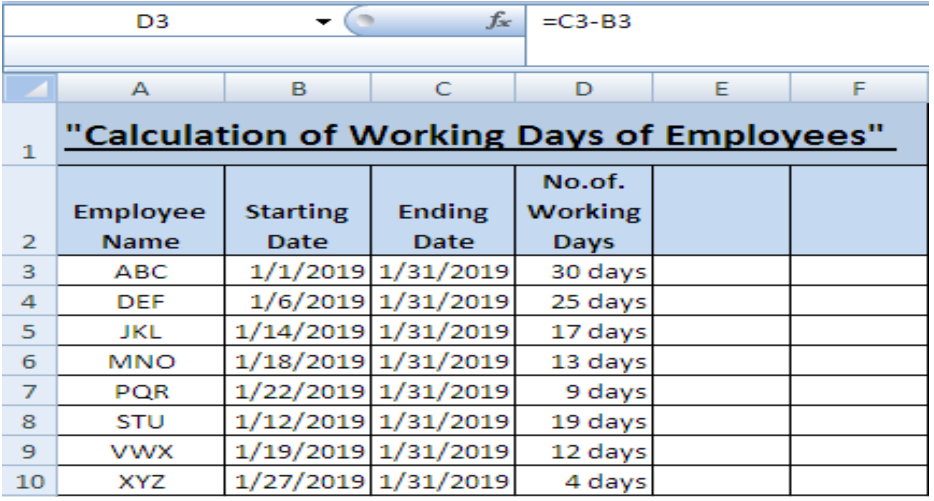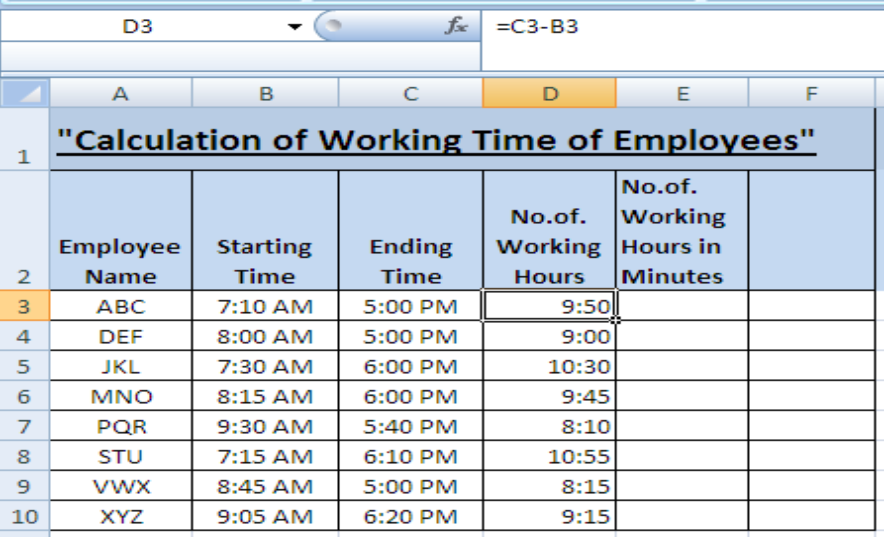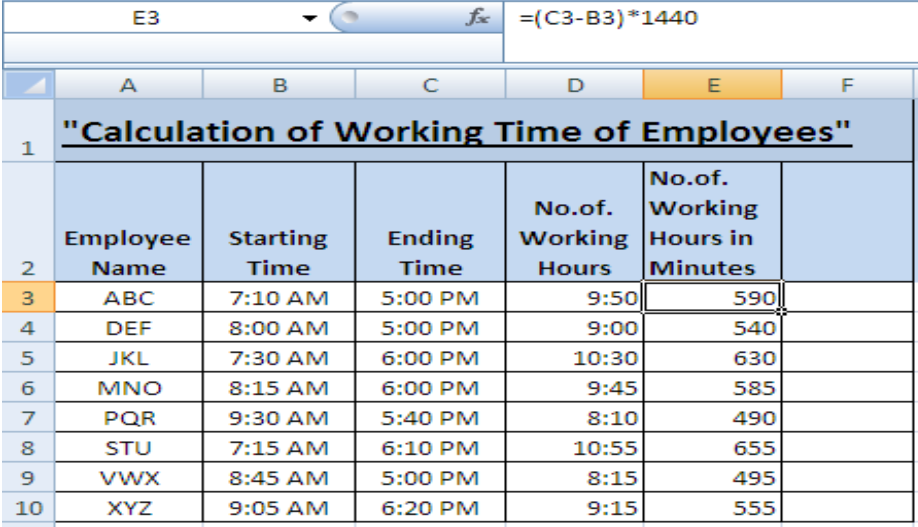Get instant live expert help with Excel or Google Sheets“My Excelchat expert helped me in less than 20 minutes, saving me what would have been 5 hours of work!”

#### Post your problem and you’ll get Expert help in seconds.

Your message must be at least 40 characters
Our professional Expert are available now. Your privacy is guaranteed.

# Learn How to Calculate Between Two Times in Excel

This article contains the information regarding calculation of time difference between two different times in Excel.

We are going to learn about how to calculate the number of days, hours and minutes between two times with the help of simplest formulas in the given examples:

## Example 1Figure 1. Example 1

Calculation of Days between two dates: This data carries supposed employees and their starting and ending dates of work in order to calculate their “total working days”.

Formula: `=Ending date-Starting date`

• Select cells from B3 to C10, make a right click and select “format cells” and a window will appear.
• Click on “date” in the “number” section and choose one specific format of date for both columns B and C.
• Click on cell D3 and enter the formula =C3-B3 and you’ll get the difference of dates that is total number of working days.
• Drag it to D10 to calculate the days between all the times.

(If we want to mention the word “days” along the numbers, Select cells from D3 to D10 and click “custom” in the format cells window and write d “days” in the “type” bar)

This feature is optional to add.

## Example 2Figure 2. Example 2

Calculation of Hours between two times: A similar figure of example is taken here to calculate the difference of hours between two times.

Formula: `=Ending time-Starting time`

• Select cells B3 to C10, press a shortcut key CTRL+1 for the format cell window and choose the format from “time” in the “number” section.
• Select cell D3 to D10 and format them by clicking on h:mm in the custom category of format cells window.
• Type `=C3-B3` in cell D3 and we’ll get 9:50 as total working hours (In which 9 is referred as hours whereas 50 is the number of minutes.
• Drag it to D10 to calculate between other times in columns C and B.

## Example 3Figure 3. Example 3

Calculation of Minutes between two times: In order to calculate the working time in minutes. We can follow a simple formula. That is:

Formula: `=(Ending time-Starting time)*1440`

• Click on cell E3 and follow the given formula to calculate the difference of time in minutes.
• We can see the formula entered in E3 in the formula bar.
• Click E3 and drag it down to E10 to get the working time in minutes for all the cells.

Most of the time, the problem you will need to solve will be more complex than a simple application of a formula or function. If you want to save hours of research and frustration, try our live Excelchat service! Our Excel Experts are available 24/7 to answer any Excel question you may have. We guarantee a connection within 30 seconds and a customized solution within 20 minutes.